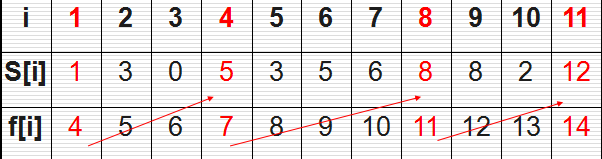### 活动选择问题理解贪心算法

{a3,a9,a11}是一个兼容的活动子集，但它不是最大子集，因为子集{a1,a4,a8,a11}更大，实际上它是我们这个问题的最大兼容子集，但它不是唯一的一个{a2，a4，a9，a11}1.动态规划算法

```       static void Main(string[] args)
{
//添加两个活动，一个从0点到0点，一个从24点到24点
//记录活动start时间的数组
int[] s = { 0, 1, 3, 0, 5, 3, 5, 6, 8, 8, 2, 12, 24 };
//记录活动end时间的数组
int[] e = { 0, 4, 5, 6, 7, 8, 9, 10, 11, 12, 13, 14, 24 };
//记录所有方案的二维数组
//数组的行数和列数对应了活动方案的编号，如result[3,7]代表了第3个到第7个活动的活动最大兼容子集
List<int>[,] result = new List<int>[13, 13];
//为二维数组赋初值，集合中的数字代表哪些活动组合成最大兼容子集
for (int m = 0; m < 13; m++)
{
for (int n = 0; n < 13; n++)
{
result[m, n] = new List<int>();
}
}
//双重循环
//依次遍历存储所有活动的最大兼容子集，j是最后一个活动编号，i是第一个活动编号
for (int j = 0; j < 13; j++)
{
for (int i = 0; i < j - 1; i++)
{
//int集合sij用于存储计算出的第i到第j个活动的最大兼容子集
List<int> sij = new List<int>();
//循环遍历每一个活动，number是当前指向的活动编号
for (int number = 1; number < s.Length - 1; number++)
{
//如果当前遍历到的活动的时间在最后一个活动j的开始时间和第一个活动i的结束时间之间，这个活动就能插入两个活动之间进行
if (s[number] >= e[i] && e[number] <= s[j])
{
sij.Add(number);
}
}
//如果有活动插入到了两个活动之间
if (sij.Count > 0)
{
//保存最大兼容子集的活动的数量
int maxCount = 0;
//保存最大兼容子集
List<int> tempList = new List<int>();
//循环遍历插入的活动
foreach (int number in sij)
{
//计算最大兼容子集的活动的数量
int count = result[i, number].Count + result[number, j].Count + 1;
//更新最大兼容子集的活动数量
//可能有多个活动可以插入两个活动之间，因此需要判断哪一个活动插入后是从i活动到j活动的最大兼容子集
//将最大兼容子集的活动编号保存起来
if (maxCount < count)
{
maxCount = count;
tempList = result[i, number].Union<int>(result[number, j]).ToList<int>();
tempList.Add(number);
}
}
//更新计算出的i活动到j活动的最大兼容子集
result[i, j] = tempList;
}
}
}
//结果取出第0个活动到第12个活动的最大兼容子集即可
List<int> a = result[0, 12];
foreach (int item in a)
{
Console.Write(item + " ");
}
Console.ReadKey();
}```

2.贪心算法（递归解决）

```        static void Main(string[] args)
{
List<int> list = ActivitySelection(1, 11, 0, 24);
foreach(int t in list)
{
Console.Write(t + " ");
}
Console.ReadKey();
}
static int[] s = { 0, 1, 3, 0, 5, 3, 5, 6, 8, 8, 2, 12, 24 };
static int[] e = { 0, 4, 5, 6, 7, 8, 9, 10, 11, 12, 13, 14, 24 };
/// <summary>
/// 计算给定的活动编号之间的活动能否在给定时间内进行
/// </summary>
/// <param name="startActivityNumber">开始的活动编号</param>
/// <param name="endActivityNumber">结束的活动编号</param>
/// <param name="startTime">开始时间</param>
/// <param name="endTime">结束时间</param>
/// <returns></returns>
public static List<int> ActivitySelection(int startActivityNumber,int endActivityNumber,int startTime,int endTime)
{
//如果活动已经找完或者时间已经找完，结束递归调用
if (startActivityNumber > endActivityNumber || startTime >= endTime)
return new List<int>();
//记录可以插入的活动编号
int tempNumber = 0;
//循环遍历活动编号，看能否在给定时间内进行
for (int number = startActivityNumber; number <= endActivityNumber; number++)
{
//判断能否在给定时间内进行
if(s[number] >= startTime && e[number] <= endTime)
{
tempNumber = number;
break;
}
}
//找到了一个能插入的活动后，继续递归找下一个能插入的活动
List<int> list = ActivitySelection(tempNumber + 1, endActivityNumber, e[tempNumber], endTime);
//将找到的活动编号添加入集合中
list.Add(tempNumber);
//返回找到的结果
return list;
}```

3.贪心算法（迭代解决）

```        static void Main(string[] args)
{
List<int> list = ActivitySelection(1, 11, 0, 24);
foreach(int t in list)
{
Console.Write(t + " ");
}
Console.ReadKey();
}
static int[] s = { 0, 1, 3, 0, 5, 3, 5, 6, 8, 8, 2, 12, 24 };
static int[] e = { 0, 4, 5, 6, 7, 8, 9, 10, 11, 12, 13, 14, 24 };
/// <summary>
/// 计算给定的活动编号之间的活动能否在给定时间内进行
/// </summary>
/// <param name="startActivityNumber">开始的活动编号</param>
/// <param name="endActivityNumber">结束的活动编号</param>
/// <param name="startTime">开始时间</param>
/// <param name="endTime">结束时间</param>
/// <returns></returns>
public static List<int> ActivitySelection(int startActivityNumber,int endActivityNumber,int startTime,int endTime)
{
//保存结果的list
List<int> list = new List<int>();
//循环遍历活动编号，看能否在给定时间内进行
for (int number = 1; number <= 11; number++)
{
//判断能否在给定时间内进行，找到可以在给定时间内进行的活动后将活动编号加入结果的集合，并且下一个活动的开始时间需要更新
if(s[number] >= startTime && e[number] <= endTime)
{
list.Add(number);
startTime = e[number];
}
}
//返回找到的结果
return list;
}```